Next: About the Software Examples Up: Sinusoids, amplitude and frequency Previous: Superposing Signals   Contents   Index

# Periodic Signals

A signal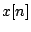is said to repeat at a period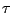iffor all. Such a signal would also repeat at periods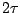and so on; the smallestif any at which a signal repeats is called the signal's period. In discussing periods of digital audio signals, we quickly run into the difficulty of describing signals whose period" isn't an integer, so that the equation above doesn't make sense. For now we'll effectively ignore this difficulty by supposing that the signalmay somehow be interpolated between the samples so that it's well defined whetheris an integer or not.

A sinusoid has a period (in samples) ofwhereis the angular frequency. More generally, any sum of sinusoids with frequencies, for integers, will repeat aftersamples. Such a sum is called a Fourier Series:Moreover, if we make certain technical assumptions (in effect that signals only contain frequencies up to a finite bound), we can represent any periodic signal as such a sum. This is the discrete-time variant of Fourier analysis which will reappear in Chapter 9.

The angular frequencies of the sinusoids above are all integer multiples of. They are called the harmonics of, which in turn is called the fundamental. In terms of pitch, the harmonics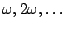are at intervals of 0, 1200, 1902, 2400, 2786, 3102, 3369, 3600, ..., cents above the fundamental; this sequence of pitches is sometimes called the harmonic series. The first six of these are all quite close to multiples of 100; in other words, the first six harmonics of a pitch in the Western scale land close to (but not always exactly on) other pitches of the same scale; the third and sixth miss only by 2 cents and the fifth misses by 14.

Put another way, the frequency ratio 3:2 (a perfect fifth in Western terminology) is almost exactly seven half-steps, 4:3 (a perfect fourth) is just as near to five half-steps, and the ratios 5:4 and 6:5 (perfect major and minor thirds) are fairly close to intervals of four and three half-steps, respectively.

A Fourier series (with only three terms) is shown in Figure 1.8. The first three graphs are of sinusoids, whose frequencies are in a 1:2:3 ratio. The common period is marked on the horizontal axis. Each sinusoid has a different amplitude and initial phase. The sum of the three, at bottom, is not a sinusoid, but it still maintains the periodicity shared by the three component sinusoids.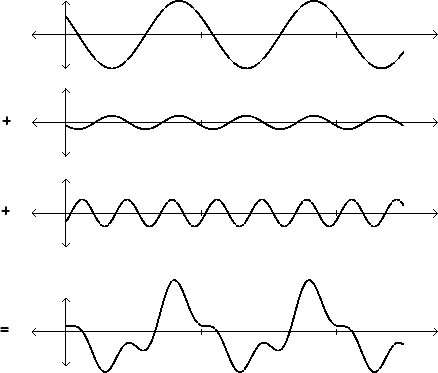Leaving questions of phase aside, we can use a bank of sinusoidal oscillators to synthesize periodic tones, or even to morph smoothly through a succession of periodic tones, by specifying the fundamental frequency and the (possibly time-varying) amplitudes of the partials. Figure 1.9 shows a block diagram for doing this.This is an example of additive synthesis; more generally the term can be applied to networks in which the frequencies of the oscillators are independently controllable. The early days of computer music rang with the sound of additive synthesis.Next: About the Software Examples Up: Sinusoids, amplitude and frequency Previous: Superposing Signals   Contents   Index
Miller Puckette 2006-12-30